# Difference between revisions of "Levi-Civita connection"

This lives as an element of: the space of all linear connections, which in turn sits inside the space of all$\R$-bilinear maps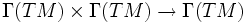$\Gamma(TM) \times \Gamma(TM) \to \Gamma(TM)$

## Definition

### Given data

A Riemannian manifold$(M,g)$ (here,$M$ is a differential manifold and$g$ is the additional structure of a Riemannian metric on it).

More generally, we can also look at a pseudo-Riemannian manifold, or a manifold with a pseudo-Riemannian metric: a smoothly varying nondegenerate (not necessarily positive definite) symmetric bilinear form$g$ in each tangent space.

### Definition part

A Levi-Civita connection on$(M,g)$ is a linear connection$\nabla$ on$M$ satisfying the following two conditions:

• The connection is metric, viz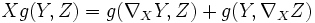$X g (Y,Z) = g (\nabla_X Y, Z) + g(Y, \nabla_X Z)$
• The connection is torsion-free, viz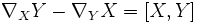$\nabla_X Y - \nabla_Y X = [X,Y]$.

### Definition by formula

The formula for the Levi-Civita connection requires us to use the fact that$g$ is nondegenerate. So, instead of directly specifying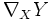$\nabla_XY$ for vector fields$X$ and$Y$, the formula specifies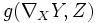$g(\nabla_XY,Z)$ for$X,Y,Z$ vector fields, as follows: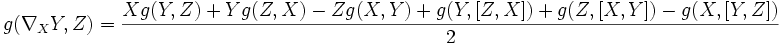$g(\nabla_XY,Z) = \frac{Xg(Y,Z) + Yg(Z,X) - Zg(X,Y) + g(Y,[Z,X]) + g(Z,[X,Y]) - g(X,[Y,Z])}{2}$.

## Facts

### The Levi-Civita connection is unique

Further information: Levi-Civita connection exists and is unique

The proof combines the metric condition, the torsion-free condition, and the nondegeneracy of$g$.

Note that the nondegeneracy of$g$ is very important, otherwise knowing the value of the inner product for any three vectors may not necessarily help us in computing the value of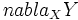$nabla_XY$

To show that the Levi-Civita connection exists, it suffices to check that the map sending$Z$ to what we propose for$g(\nabla_X Y, Z)$ is actually a linear map.

### Christoffel symbols

Further information: Christoffel symbol

The Levi-Civita connection on a manifold$M$ is a map$\nabla: \Gamma(TM) \times \Gamma(TM) \to \Gamma(TM)$. This means that at any point$p \in M$, it gives a map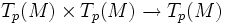$T_p(M) \times T_p(M) \to T_p(M)$, which roughly differentiates one tangent vector along another.

Let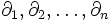$\partial_1, \partial_2, \ldots, \partial_n$ form a basis for the tangent space$TM$. Then, the Christoffel symbol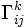$\Gamma_{ij}^k$ is the component along$e_k$ of the vector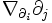$\nabla_{\partial_i}\partial_j$.

The Christoffel symbols thus give an explicit description of the Levi-Civita connection. Namely, the Levi-Civita connection can be expressed using the Christoffel symbols.